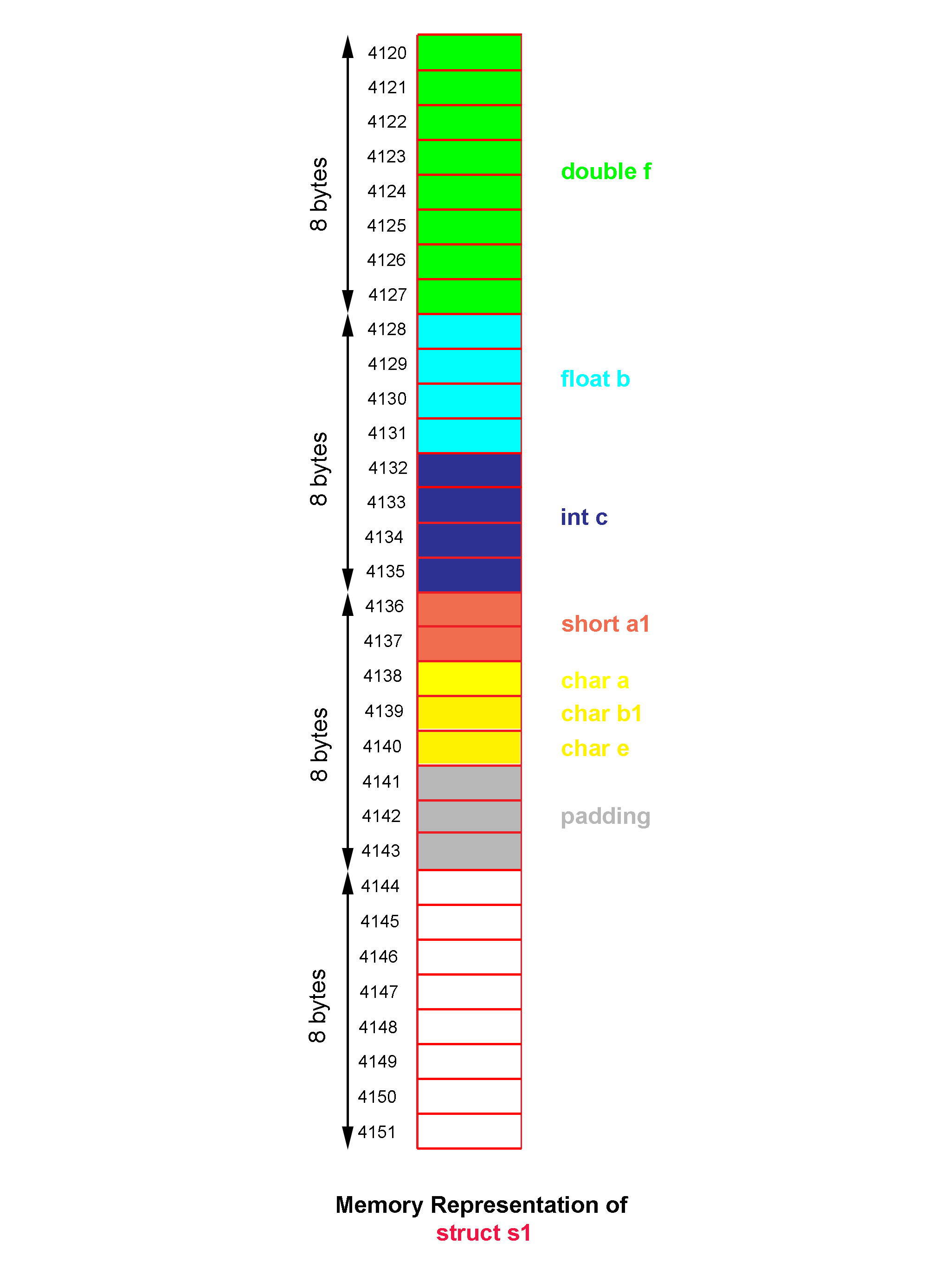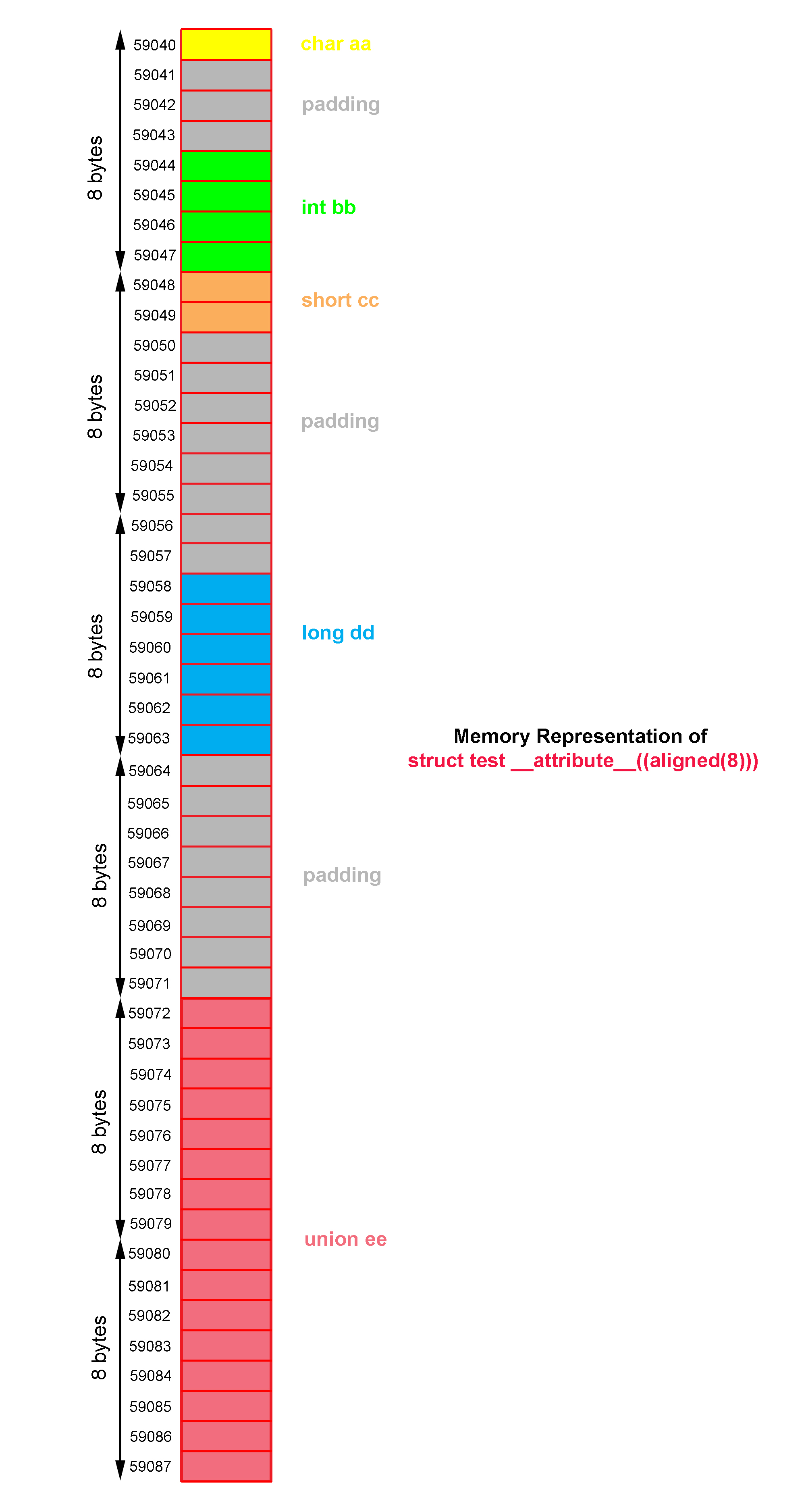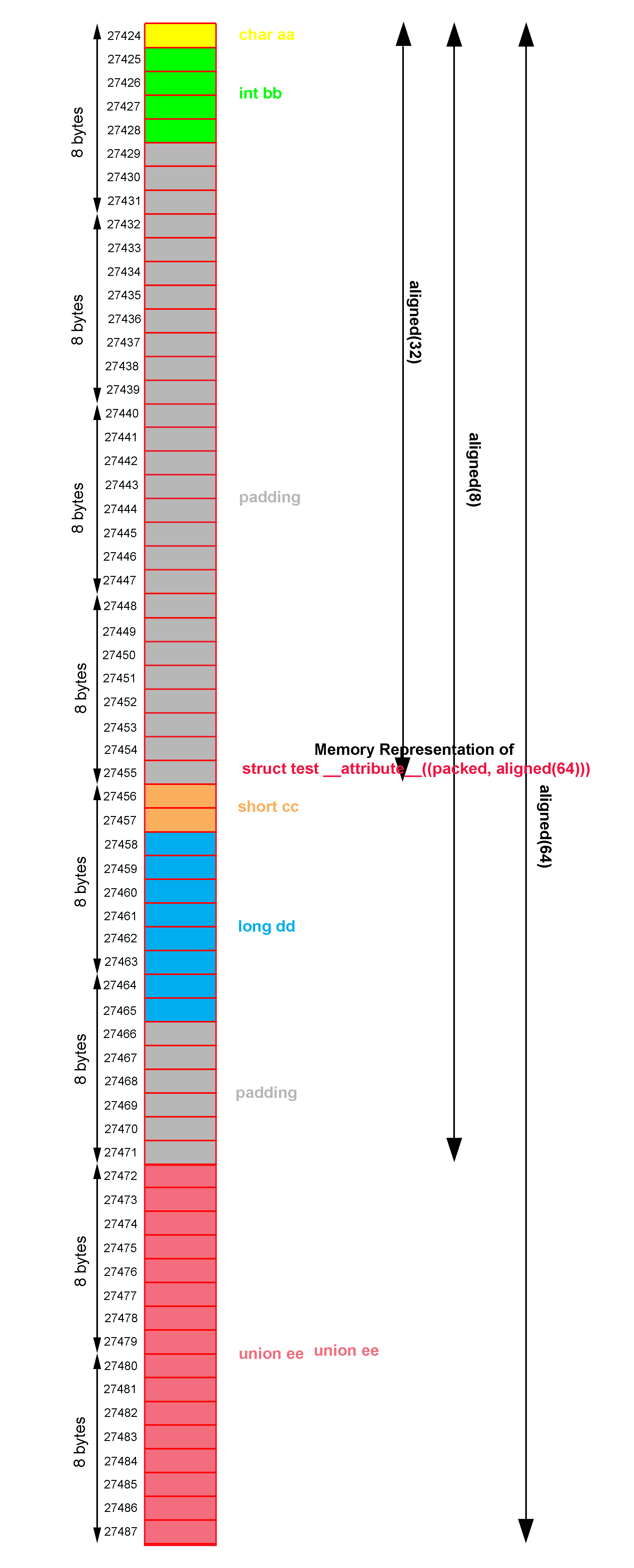Structure Alignment and Packing in C Programming

July 06, 2018 | 1 Tags | 0 CommentA modern CPU fetches data from memory in multiples of word sized chunks. The word size is dependent on the platform. For 64-bit platform, it would be 8-bytes, meaning that the CPU would fetch data in multiples of 8. For 32-bit platform, the word size is 4-bytes.

The storage for data does not normally start at arbitrary byte addresses in memory. Aligning data in memory to be in multiples of word size helps the read performance.

Every data type has an alignment associated with it which is mandated by the processor architecture rather than the language itself. Aligning data elements allows the processor to fetch data from memory in an efficient manner and thereby improves the performance. The compiler tries to maintain these alignments for data elements to provide optimal performance. The typical alignment requirement for data types on 32-bit and 64-bit platform are shown below

Data Type 32-bit (bytes) 64-bit (bytes)
char 1 1
short 2 2
int 4 4
long 8 8
float 4 4
double 8 8
long long 8 8
long double 4 16
Any pointer 4 8

For structures that generally contain data elements of different types, the compiler tries to maintain the proper alignment of data elements by inserting unused memory between elements. This technique known as Padding. The compiler also aligns the entire structure to its most strictly aligned member. The compiler may also increase the size of structure if necessary, to make it a multiple of the alignment by adding padding at the end of the structure. This is known as Tail Padding.

Let’s say we have a 64-bit platform and we create a structure like the one below

struct my_struct {
char *p;  /* 8 bytes */
char c;   /* 1 byte */
long x;   /* 8 bytes */
};

You might think that sizeof(struct my_struct) should be 10 bytes, but it’s actually 24 bytes. This is because of self-alignment. Compiler inserted 7 bytes of padding between c and x to keep the structure aligned.

struct my_struct {
char *p;  /* 8 bytes */
char c;   /* 1 byte */
char padding;   /* 7 bytes */
long x;   /* 8 bytes */
};You can minimize the memory wastage by ordering the structure elements such that the widest (largest) element comes first, followed by the second widest, and so on. The following example helps illustrate the effect of ordering of data elements on the size of the structure.

struct s1
{
char a;  /* 1 byte */
short a1;   /* 2 bytes */
char b1;   /* 1 byte */
float b;   /* 4 bytes */
int c;   /* 4 bytes */
char e;   /* 1 byte */
double f;   /* 8 bytes */
};

The structure above can be aligned and padded to be as follows

struct s1
{
char a;  /* 1 byte */
char pad1; /* 1 byte */
short a1;   /* 2 bytes */
char b1;   /* 1 byte */
char pad2; /* 3 bytes */
float b;   /* 4 bytes */
int c;   /* 4 bytes */
char e;   /* 1 byte */
char pad3;  /* 7 bytes */
double f;   /* 8 bytes */
};The size of struct s1 after being aligned and padded is 32 bytes.

Now we re-arrange the structure as follows

struct s1
{
double f;
float b;
int c;
short a1;
char a,b1,e;
};

After being aligned and padded, the structure will be as follows

struct s1
{
double f;
float b;
int c;
short a1;
char a;
char b1;
char e;
};The size of the struct s1 after re-arranging the elements is 24 bytes. Hence, simply re-arranging the elements during the structure definition may help in avoiding memory wastage.

You can specify the structure members in bits. Structures in C are capable of implementing bit fields. Bit fields allows us to access particular fields stored in an integer. Floating point types are not allowed as bit fields.

For example, we want to store a date with the details of date, month and year.

struct date
{
short dt;
short mn;
short yr;
};

struct date today;

The size of variable today of struct date type is 6 bytes (48 bits). We don’t need this much memory. It is enough to have just 23 bits of memory that is 6 bits to store maximum date of 31, 5 bits to store maximum month of 12, and 12 bits to store maximum year of 2047.

The members of struct date are signed types, member dt can hold value from -32 to +31, member mn can hold value from -16 to +15, and member yr can hold value from -2048 to +2047. Actually we don’t need the signed value. Hence we can further save the memory by defining the bit fields as unsigned type.

#include<stdio.h>

struct date
{
unsigned short dt:5;
unsigned short mn:4;
unsigned short yr:11;
};

int main()
{
struct date a;
a.dt=29;
a.mn=6;
a.yr=2018;

printf("Date: %u/%u/%u\n", a.dt, a.mn, a.yr);
printf("Memory Taken %lu bytes\n", sizeof(a));

return 0;
}

Output:

Date: 29/6/2018
Memory Taken 4 bytes

Generally, bytes have address but bits have no address, hence the operator & cannot be used to access the address of bit fields. Trying to access the address of bit fields result compilation error.

#include<stdio.h>

struct date
{
unsigned short dt:5;
unsigned short mn:4;
unsigned short yr:11;
};

int main()
{
struct date a;

printf("%u\n%u\n%u\n", &a.dt, &a.mn, &a.yr);

return 0;
}

Output:

example.c: In function 'main':
example.c:11:2: error: cannot take address of bit-field 'dt'
printf("%u\n%u\n%u",&a.dt,&a.mn,&a.yr);
^
example.c:11:2: error: cannot take address of bit-field 'mn'
example.c:11:2: error: cannot take address of bit-field 'yr'

A bit field can’t be defined with the size more than its data size. For example, a bit can’t be defined as

unsigned short x:17;

Because under any platform the size of short type is 2 bytes or 16 bits, specifying more than 16 bits is illegal and results compilation error.

You can use pragma directive to specify the new alignment in bytes for structures.

#include<stdio.h>

struct test {
char  aa;    // size = 1
int   bb;    // size = 4
short cc;    // size = 2
long  dd;    // size = 8
union {      // size = 16 (_Decimal128 is 16, using union to set the value)
_Decimal128 ee1;
long ee2;
} ee;
};
int main()
{
struct test a;
a.aa=(char)0x44;
a.bb=0x55555555;
a.cc=0x6666;
a.dd=0x7777777777777777;
a.ee.ee2=0x8888888888888888;
a.ee.ee2=0x8888888888888888;

printf("Size of product: %lu\n", sizeof(a));

return 0;
}

Output:

Size of product: 48

#pragma pack(n) option (n=1,2,4,8 or 16) allows you to change the alignment of data types within a struct to align to boundaries smaller than its size. It doesn’t force alignment of ALL variables, it only changes the alignment of variables larger than the pack setting. For example, if we set #pragma pack to 8, the 16-byte _Decimal128 data type aligns on the 8-byte boundary, but the other data types still use their natural alignment.

#include<stdio.h>
#pragma pack(8)

struct test {
char  aa;    // size = 1
int   bb;    // size = 4
short cc;    // size = 2
long  dd;    // size = 8
union {      // size = 16 (_Decimal128 is 16, using union to set the value)
_Decimal128 ee1;
long ee2;
} ee;
};
int main()
{
struct test a;
a.aa=(char)0x44;
a.bb=0x55555555;
a.cc=0x6666;
a.dd=0x7777777777777777;
a.ee.ee2=0x8888888888888888;
a.ee.ee2=0x8888888888888888;

printf("Size of struct test: %lu\n", sizeof(a));

return 0;
}

Output:

Size of struct test: 40

How about if we change the pack setting to 4? Now both 8-byte variable dd and 16-byte variable ee have changed alignment.

#include<stdio.h>
#pragma pack(4)

struct test {
char  aa;    // size = 1
int   bb;    // size = 4
short cc;    // size = 2
long  dd;    // size = 8
union {      // size = 16 (_Decimal128 is 16, using union to set the value)
_Decimal128 ee1;
long ee2;
} ee;
};
int main()
{
struct test a;
a.aa=(char)0x44;
a.bb=0x55555555;
a.cc=0x6666;
a.dd=0x7777777777777777;
a.ee.ee2=0x8888888888888888;
a.ee.ee2=0x8888888888888888;

printf("Size of struct test: %lu\n", sizeof(a));

return 0;
}

Output:

Size of struct test: 36

Continuing on to pack setting of 2

#include<stdio.h>
#pragma pack(2)

struct test {
char  aa;    // size = 1
int   bb;    // size = 4
short cc;    // size = 2
long  dd;    // size = 8
union {      // size = 16 (_Decimal128 is 16, using union to set the value)
_Decimal128 ee1;
long ee2;
} ee;
};
int main()
{
struct test a;
a.aa=(char)0x44;
a.bb=0x55555555;
a.cc=0x6666;
a.dd=0x7777777777777777;
a.ee.ee2=0x8888888888888888;
a.ee.ee2=0x8888888888888888;

printf("Size of struct test: %lu\n", sizeof(a));

return 0;
}

Output:

Size of struct test: 32

At pack setting of 1, there are no more padding bytes and the struct is as small as possible.

#include<stdio.h>
#pragma pack(1)

struct test {
char  aa;    // size = 1
int   bb;    // size = 4
short cc;    // size = 2
long  dd;    // size = 8
union {      // size = 16 (_Decimal128 is 16, using union to set the value)
_Decimal128 ee1;
long ee2;
} ee;
};
int main()
{
struct test a;
a.aa=(char)0x44;
a.bb=0x55555555;
a.cc=0x6666;
a.dd=0x7777777777777777;
a.ee.ee2=0x8888888888888888;
a.ee.ee2=0x8888888888888888;

printf("Size of struct test: %lu\n", sizeof(a));

return 0;
}

Output:

Size of product: 31

If we want to set the new alignment only on a certain struct, we can use #pragma pack(push,n) and #pragma pack(pop) where n is 1, 2, 4, 8 or 16.

#include<stdio.h>

struct test1 {
char  aa;    // size = 1
int   bb;    // size = 4
short cc;    // size = 2
long  dd;    // size = 8
union {      // size = 16 (_Decimal128 is 16, using union to set the value)
_Decimal128 ee1;
long ee2;
} ee;
};

#pragma pack(push,1)
struct test2 {
char  aa;    // size = 1
int   bb;    // size = 4
short cc;    // size = 2
long  dd;    // size = 8
union {      // size = 16 (_Decimal128 is 16, using union to set the value)
_Decimal128 ee1;
long ee2;
} ee;
};
#pragma pack(pop)

int main()
{
struct test1 a;
a.aa=(char)0x44;
a.bb=0x55555555;
a.cc=0x6666;
a.dd=0x7777777777777777;
a.ee.ee2=0x8888888888888888;
a.ee.ee2=0x8888888888888888;

struct test2 b;
b.aa=(char)0x44;
b.bb=0x55555555;
b.cc=0x6666;
b.dd=0x7777777777777777;
b.ee.ee2=0x8888888888888888;
b.ee.ee2=0x8888888888888888;

printf("Size of struct test1: %lu\n", sizeof(a));

printf("Size of struct test2: %lu\n", sizeof(b));

return 0;
}

Output:

Size of struct test1: 48
Size of struct test2: 31

You may also use attributes to specify a new alignment.

• __attribute__((aligned(n))) , this attribute sets the minimum alignment of the specified variables to a specific number of bytes. n must be a the positive of 2 or NIL. NIL can be specified as either __attribute__((aligned())) or __attribute__((aligned)), the compiler automatically sets the alignment for the declared variable or field to the largest alignment
• __attribute__((packed)) , this attribute specifies that a variable or structure field should have the smallest possible alignment – one byte for a variable and one bit for a field
#include<stdio.h>

struct test {
char  aa;    // size = 1
int   bb;    // size = 4
short cc;    // size = 2
long  dd;    // size = 8
union {      // size = 16 (_Decimal128 is 16, using union to set the value)
_Decimal128 ee1;
long ee2;
} ee;
} __attribute__ ((aligned (8)));

int main()
{
struct test a;
a.aa=(char)0x44;
a.bb=0x55555555;
a.cc=0x6666;
a.dd=0x7777777777777777;
a.ee.ee2=0x8888888888888888;
a.ee.ee2=0x8888888888888888;

printf("Size of struct test: %lu\n", sizeof(a));

return 0;
}
Size of struct test: 48The structure declaration above forces the compiler to ensure that each variable of type struct test is aligned on a 8-byte boundary and the size of variables of type struct test should be a multiple of 8 bytes.

#include<stdio.h>

struct test {
char  aa;
int   bb;
short cc __attribute__ ((aligned (32)));
long  dd;
union {
_Decimal128 ee1;
long ee2;
} ee __attribute__ ((aligned (8)));
} __attribute__ ((aligned (64)));

int main()
{
struct test a;
a.aa=(char)0x44;
a.bb=0x55555555;
a.cc=0x6666;
a.dd=0x7777777777777777;
a.ee.ee2=0x8888888888888888;
a.ee.ee2=0x8888888888888888;

printf("Size of struct test: %lu\n", sizeof(a));

return 0;
}
Size of struct test: 64The structure declaration above ensure that each variable of type struct test is aligned on a 8-byte boundary and the size of variables of type struct test should be a multiple of 64 bytes.

Having attribute __attribute__ ((aligned (32))) on variable cc makes the variable aa and bb aligned on a 32-byte boundary and the total size of those variables should be a multiple of 32 bytes.

Having attribute __attribute__ ((aligned (8))) on union ee makes the variable aa, bb, cc, and dd aligned on a 8-byte boundary and the total size of those variables should be a multiple of 8 bytes.

#include<stdio.h>

struct test {
char  aa;
int   bb;
short cc;
long  dd;
union {
_Decimal128 ee1;
long ee2;
} ee;
} __attribute__ ((packed, aligned (64)));

int main()
{
struct test a;
a.aa=(char)0x44;
a.bb=0x55555555;
a.cc=0x6666;
a.dd=0x7777777777777777;
a.ee.ee2=0x8888888888888888;
a.ee.ee2=0x8888888888888888;

printf("Size of struct test: %lu\n", sizeof(a));

return 0;
}
Size of struct test: 64Having attribute __attribute__ ((packed)) on struct test makes its each member aligned on a 1-byte boundary.

Having attribute __attribute__ ((aligned(64))) on struct test makes the size of variables of type struct test should be a multiple of 64 bytes.

#include<stdio.h>

struct test {
char  aa;
int   bb;
short cc __attribute__ ((aligned (32)));
long  dd;
union {
_Decimal128 ee1;
long ee2;
} ee __attribute__ ((aligned (8)));
} __attribute__ ((packed, aligned (64)));

int main()
{
struct test a;
a.aa=(char)0x44;
a.bb=0x55555555;
a.cc=0x6666;
a.dd=0x7777777777777777;
a.ee.ee2=0x8888888888888888;
a.ee.ee2=0x8888888888888888;

printf("Size of struct test: %lu\n", sizeof(a));

return 0;
}
Size of struct test: 64Having attribute __attribute__ ((packed)) on struct test makes its each member aligned on a 1-byte boundary.

Having attribute __attribute__ ((aligned(64))) on struct test makes the size of variables of type struct test should be a multiple of 64 bytes.

Having attribute __attribute__ ((aligned (32))) on variable cc makes the total size of variable aa and bb should be a multiple of 32 bytes.

Having attribute __attribute__ ((aligned (8))) on union ee makes the total size of variable aa, bb, cc, and dd should be a multiple of 8 bytes.Samuel Yang

If you like this tutorial, you can support me